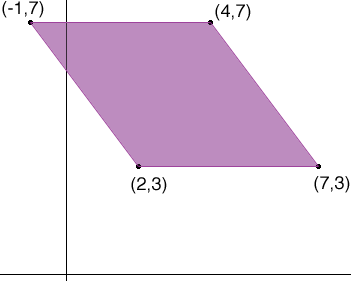SEARCH HOMEMath Central Quandaries & QueriesQuestion from Matthew, a student: Quadrilateral KLMN has vertices K(2,3), L(7,3), M(4,7) and N(-1,7). How do I prove that the quadrilateral is a Rhombus?Matthew,

A rhombus is a quadrilateral with all sides equal in length.

Carefully draw a diagram.Are the sides of equal length? Why or why not?

Penny

A rhombus has opposite sides equal in length and parallel - that is, equal as vectors.

To find the side as a vector subtract one endpoint (as a vector, or coordinate pair) from the other.

Good Hunting!

RDMath Central is supported by the University of Regina and The Pacific Institute for the Mathematical Sciences.